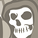# QlikView App Dev

Discussion Board for collaboration related to QlikView App Development.

Announcements
cancel
Showing results for
Did you mean:Contributor II

## Using GetFieldSelections to call values in a field

I'm very new to Qlikview, so I'm sure this question is very easy to solve. I'm creating a pivot table where the user can choose what kind of measure they want to see in the table. There are 8 different measures the user can choose from. Right now, I'm using the following syntax:

If(Measure='MYTD',[MYTD],
If(Measure='LC',[LC],
If(Measure='Rep',[Rep],
If(Measure='R',,
If(Measure='Ratio',[Ratio],
If(Measure='Spend',[Spend],
If(Measure='Wa',[Wa])))))))

Is there a way I can use GetFieldSelections to write all these in 1 line and not specify the name of each measure (in case these names change in the future)?

1 Solution

Accepted SolutionsMVP & Luminary

The suggestion from nishanthi.8 simplifies your task whereby the syntax isn't quite correct and it should be rather look like:

Pick(Match(Measure,'MYTD','LC','Rep','R','Ratio','Spend','Wa'),

[MYTD],[LC],[Rep],,[Ratio],[Spend],[Wa])

Another method - at least if your listbox-values are identically with your fieldnames - would be to use a \$-sign expansion like: [\$(=only(Measure))]

- Marcus

11 RepliesCreator

Go for pick and match. It works like this:

Pick(Match(Measure),'MYTD','LC','Rep','R',''Ratio,'Spend','Wa'))MVP

Why don't you try this... assuming that you will only select one Measure at a time

\$(=Measure)MVP & Luminary

The suggestion from nishanthi.8 simplifies your task whereby the syntax isn't quite correct and it should be rather look like:

Pick(Match(Measure,'MYTD','LC','Rep','R','Ratio','Spend','Wa'),

[MYTD],[LC],[Rep],,[Ratio],[Spend],[Wa])

Another method - at least if your listbox-values are identically with your fieldnames - would be to use a \$-sign expansion like: [\$(=only(Measure))]

- MarcusContributor II
Author

Thanks! This was helpful, but as Marcus mentioned the syntax is not complete. I need to tell the Pick function what to pick for every match.Contributor II
Author

Thanks Sunny! Should I use this within another function?Contributor II
Author

Thank you Marcus! Your pick and match function worked perfectly. I'm not sure how to use the \$ expansion though.MVP

Did you try this at all?

\$(=Measure)

or

[\$(=only(Measure))]MVP

All you need to do is to replace this

If(Measure='MYTD',[MYTD],
If(Measure='LC',[LC],
If(Measure='Rep',[Rep],
If(Measure='R',,
If(Measure='Ratio',[Ratio],
If(Measure='Spend',[Spend],
If(Measure='Wa',[Wa])))))))

with

\$(=Measure)MVP

So, for instance if you were doing this previously

Sum(

If(Measure='MYTD',[MYTD],
If(Measure='LC',[LC],
If(Measure='Rep',[Rep],
If(Measure='R',,
If(Measure='Ratio',[Ratio],
If(Measure='Spend',[Spend],
If(Measure='Wa',[Wa])))))))

)

Change it to this

Sum(\$(=Measure))